# NCERT Solutions for Class 7 Maths Chapter 2 Fractions and Decimals Ex 2.3

NCERT Solutions for Class 7 Maths Chapter 2 Fractions and Decimals Ex 2.3

### NCERT Solutions for Class 7 Maths Chapter 2 Fractions and Decimals Ex 2.3

NCERT Solutions for Class 7 Maths Chapter 2 Fractions and Decimals Exercise 2.3
Ex 2.3 Class 7 Maths Question 1.
Find:Solution:Ex 2.3 Class 7 Maths Question 2.
Multiply and reduce to lowest form (if possible):Solution: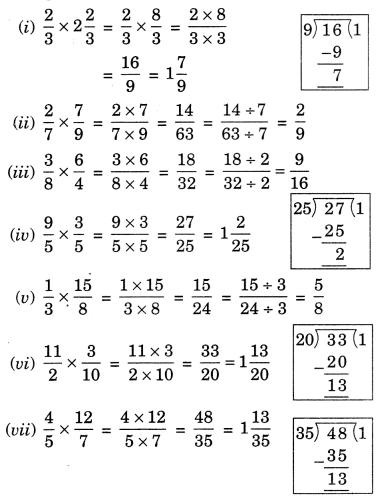Ex 2.3 Class 7 Maths Question 3.
Multiply the following fractions:Solution: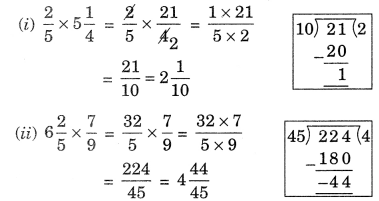Ex 2.3 Class 7 Maths Question 4.
Which is greater:Solution:Ex 2.3 Class 7 Maths Question 5.
Saili plants 4 saplings, in a row, in her garden. The distance between two adjacent saplings is 3/4 m. Find the distance between the first and the last sapling.
Solution:
Number of saplings = 4
Distance between two adjacent saplings = 3/4 m
∴ Distance between the first and the last saplingEx 2.3 Class 7 Maths Question 6.Ex 2.3 Class 7 Maths Question 7.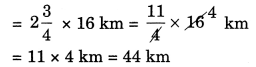Hence, the required distance = 44 km.

Ex 2.3 Class 7 Maths Question 8.Solution: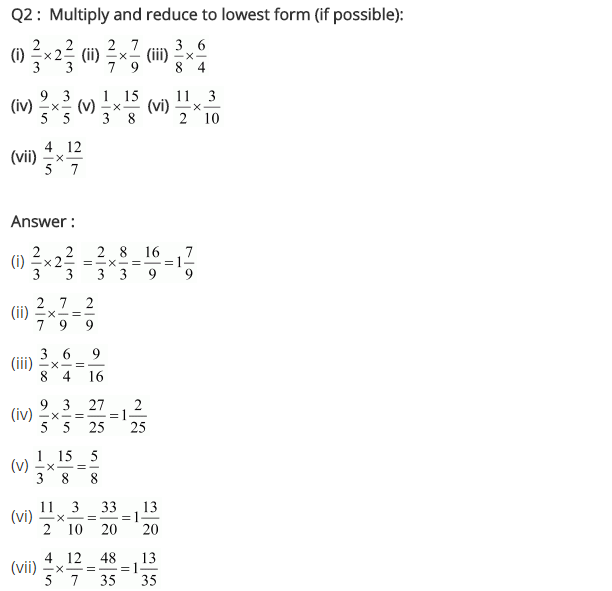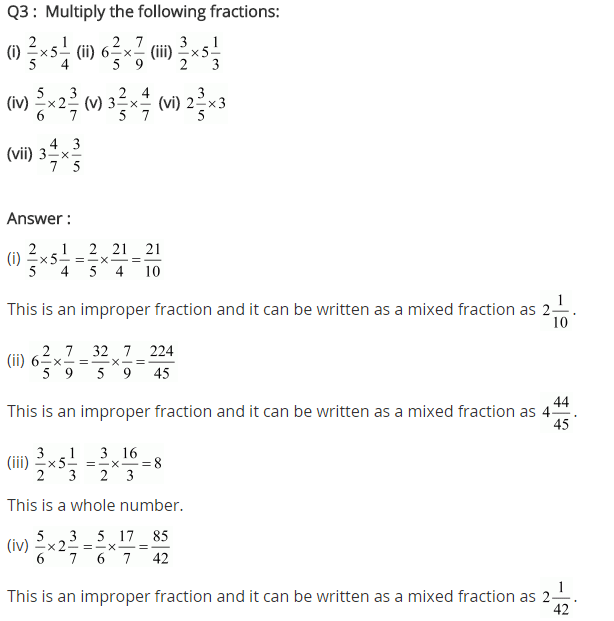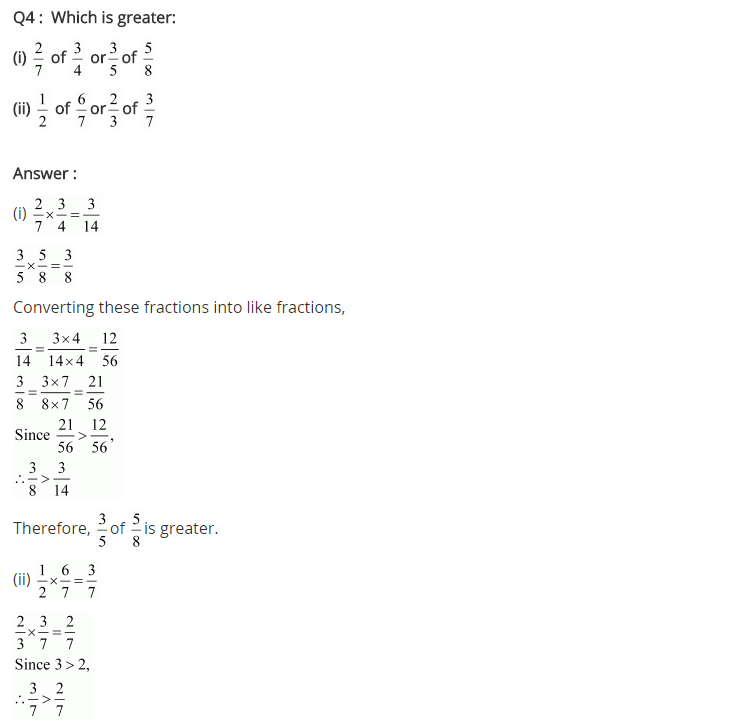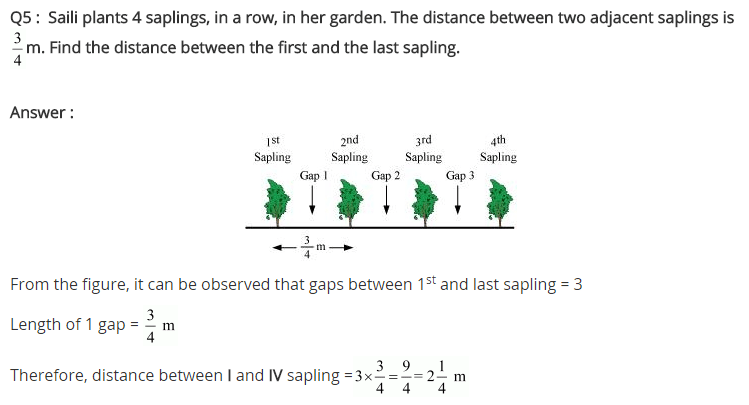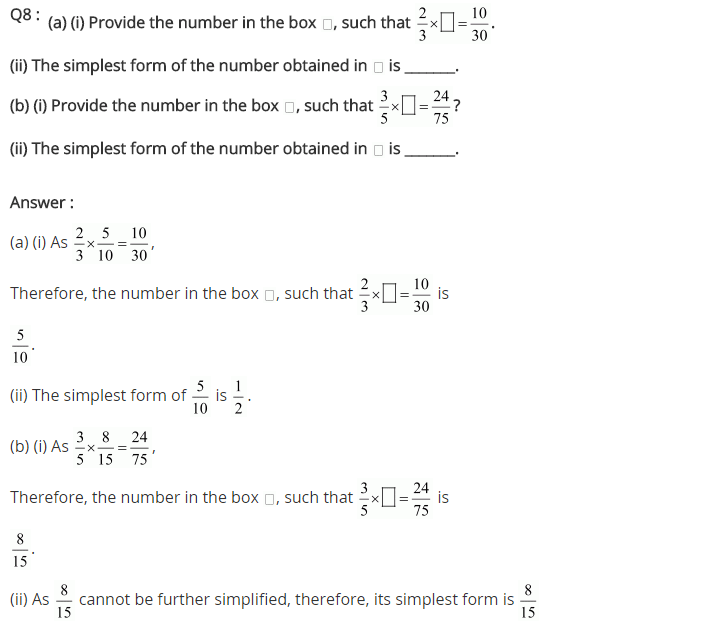## SabDekho

The Complete Educational Website